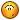BrainDen.com - Brain Teasers

## Question

The most famous Pythagorean Triangle (a right triangle with all integer sides) is the 3 , 4 , 5 where 3^2 + 4^2 = 5^2. The 'legs', a=3 and b=4, are
consecutive integers, making a nearly-isosceles right triangle. What are the next two smallest integral right triangles with consecutive legs?

## Recommended Posts

• 0

I've exhausted all triangles whose two legs differ by 1 where

the length of the shortest leg is less than or equal to 1010.

I found only 9:

3 4 5

20 21 29

119 120 169

696 697 985

4059 4060 5741

23660 23661 33461

137903 137904 195025

803760 803761 1136689

4684659 4684660 6625109

##### Share on other sites

• 0

5 12 13

Hmm do they consider the hypotenuse a leg in America?

No, they don't.##### Share on other sites

• 0

We have a^2 + b^2 = c^2; we are seeking solutions where b=a+1. So, a^2 + (a+1)^2 = c^2 => 2a^2 +2a + 1 - c^2 = 0.
Using the standard formula for solving a quadratic:
a = (1/4)*( -2 +or- sqrt(2^2 - 4*2*(1-c^2))) => a= (1/2)*(-1 + sqrt(2*c^2 - 1)) (eqn 1) [we only need pos sqrt]
For a solution in integers sqrt(2*c^2-1) is an integer, k. Then k=sqrt(2*c^2-1) => k^2 - 2*c^2 = -1.
This is a well known Pell equation which can be solved using continued fractions for sqrt(2). (see below)
Or at least, if we discover the first solution we can generate all others. First few integer solutions for (c,k) are: (1,1);(5,7);(29,41);(169,239);(985,1393);(5741,8119);(33461,47321);(195025,475807);(1136689,1607521);(6625109,9369319).
For the current problem we need only the first couple solutions (the first being trivial and the second given in the question)
Substituting the next four results into (eqn 1) gives:(c,a) = (29,20);(169,119);(985,696) and (5741,4059).
So, the required Pythag. triangles are: (a,b,c) = (20,21,29); (119,120,169);
Note:
You can generate the (a,b,c) by using m=2, n=1 ==> (3,4,5) ; then m=prev c = 5, n= prev m = 2 ==> (21,20,29), etc.
These give a ratio of successive perimeters approaching 5.828 = 3 + 2 sqrt.
This helps explain the reference to 'continued fraction solution of Pell's equation x^2 - d y^2 = +/- 1.

## Join the conversation

You can post now and register later. If you have an account, sign in now to post with your account.×   Pasted as rich text.   Paste as plain text instead

Only 75 emoji are allowed.

×   Your previous content has been restored.   Clear editor

×   You cannot paste images directly. Upload or insert images from URL.